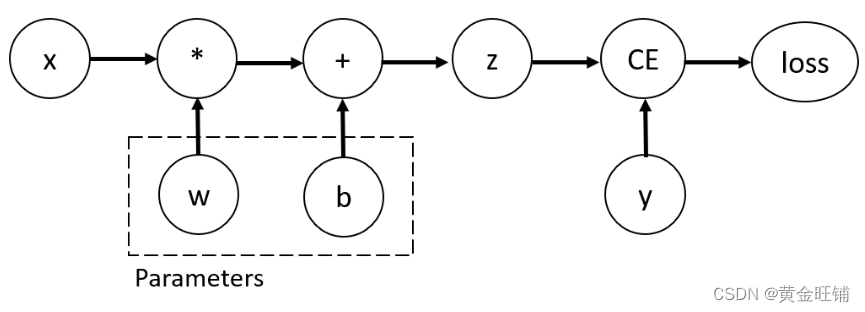news/2023/6/7 0:57:18

# AUTOMATIC DIFFERENTIATION WITH torch.autograd

import torch
x = torch.ones(5) # 输入
y = torch.zeros(3) # 输出
w = torch.randn(5, 3, requires_grad=True) # 权重
b = torch.randn(3, requires_grad=True) # 偏置
z = torch.matmul(x, w) + b
loss = torch.nn.functional.binary_cross_entropy_with_logits(z, y)
print(f"loss = {loss}")

loss = 0.7214622497558594


## Tensors, Functions and Computational graphprint(f"Gradient function for z = {z.grad_fn}")

Gradient function for z = <AddBackward0 object at 0x00000230B70C84F0>
Gradient function for loss = <BinaryCrossEntropyWithLogitsBackward object at 0x00000230B70C8D30>


loss.backward()

tensor([[0.0494, 0.0666, 0.2772],[0.0494, 0.0666, 0.2772],[0.0494, 0.0666, 0.2772],[0.0494, 0.0666, 0.2772],[0.0494, 0.0666, 0.2772]])
tensor([0.0494, 0.0666, 0.2772])


z = torch.matmul(x, w) + b

True
False


z = torch.matmul(x, w) + b
z_det = z.detach()

False


1. 将神经网络中的一些参数标记为冻结参数，这是一个非常常见的场景finetuning a pretrained network
1. 为了加速计算。因为在不跟踪梯度的 tensor 上计算会更有效。

## More on Computational Graphs

1. 根据要求的操作计算结果 tensor
2. DAG 中维护操作的梯度函数。

• 从每个 .grad_fn 中计算梯度；
• 在各自的 tensor.grad 属性中累积它们
• 利用链式法则，一直传播到叶子 tensor

PyTorch 中的 DAG 是动态的，需要注意的一件重要的事情是，图是从头开始重新创建的，在每次 .backward() 调用之后， autograd 开始填充一个新的图。 这正是允许您在模型中使用控制流语句的原因，如果需要，您可以在每次迭代中更改形状、大小和操作等。

y→=f(x→)\overrightarrow{y} = f(\overrightarrow{x}) , 当 $\overrightarrow{x}=<x_1,…x_n>$, y→=<y1,...ym>\overrightarrow{y}=<y_1,...y_m>, y→\overrightarrow{y}x→\overrightarrow{x} 的导数可以利用 Jacobian matrix (雅克比矩阵):

J=(∂y1∂x1⋯∂y1∂xn⋮⋱⋮∂ym∂x1⋯∂ym∂xn)\begin{equation*} J = \begin{pmatrix} \frac{\partial y_1}{\partial x_1} & \cdots & \frac{\partial y_1}{\partial x_n} \\ \vdots & \ddots & \vdots \\ \frac{\partial y_m}{\partial x_1} & \cdots & \frac{\partial y_m}{\partial x_n} \\ \end{pmatrix} \end{equation*}

PyTorch 允许你在给定 v=(v1,...vm)v=(v_1,...v_m) 向量时计算雅克比矩阵的点积 vT⋅Jv^T \cdot J， 而不是雅克比矩阵本身。可以通过 backwardvv 作为参数而获得。vv 的大小应该与原始的 tensor 大小相同。

inp = torch.eye(4, 5, requires_grad=True)
out = (inp+1).pow(2).t()
out.backward(torch.ones_like(out), retain_graph=True)

First call
tensor([[4., 2., 2., 2., 2.],[2., 4., 2., 2., 2.],[2., 2., 4., 2., 2.],[2., 2., 2., 4., 2.]])Second call
tensor([[8., 4., 4., 4., 4.],[4., 8., 4., 4., 4.],[4., 4., 8., 4., 4.],[4., 4., 4., 8., 4.]])Call after zeroing gradients
tensor([[4., 2., 2., 2., 2.],[2., 4., 2., 2., 2.],[2., 2., 4., 2., 2.],[2., 2., 2., 4., 2.]])


## 【参考】

Automatic Differentiation with torch.autograd — PyTorch Tutorials 1.13.1+cu117 documentation

### LINUX学习之文件处理常用命令(三)

ls 命令描述 ls命令是linux系统中用来列出文件和目录的常用命令&#xff0c;它可以显示文件和目录的名称、权限、大小、修改时间等信息 以下是ls命令的常用参数: -a &#xff1a;列出全部的文件&#xff0c;连同隐藏文件-d &#xff1a;仅列出文件目录&#xff0c;而不是列…

### 互联网乱世之下 一将功成万骨枯

&#xff08;转自虎嗅&#xff09;北京&#xff0c;入秋&#xff0c;雾霾天及社交网络的分享&#xff0c;让大家忽略了这个三千多年六朝古都传承下来的秋悲寂寥。北京的天气如二进制码般分明&#xff1a;今天重度污染&#xff0c;明天就晴空万里。而TMT行业也是如此&#xff0c…